# High School Math : Acute / Obtuse Triangles

## Example Questions

### Example Question #2 : How To Find An Angle In An Acute / Obtuse Triangle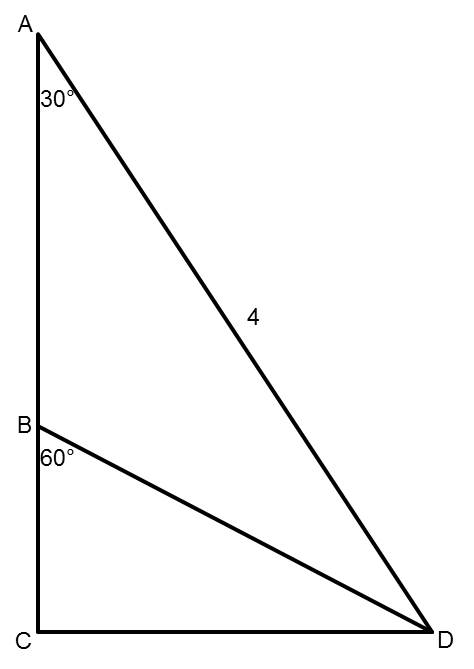Points A, B, and C are collinear (they lie along the same line). The measure of angle CAD is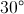. The measure of angle CBD is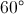. The length of segment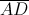is 4.

Find the measure of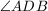.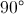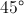Explanation:

The measure ofis. Since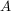,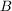, and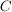are collinear, and the measure of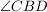is, we know that the measure of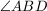is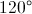.

Because the measures of the three angles in a triangle must add up to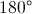, and two of the angles in triangle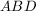areand, the third angle,, is.

### Example Question #14 : Isosceles Triangles

The base angle of an isosceles triangle is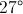.  What is the vertex angle?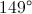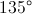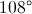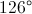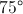Explanation:

Every triangle has 180 degrees.  An isosceles triangle has one vertex angle and two congruent base angles.

Solve the equation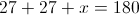for x to find the measure of the vertex angle.

x = 180 - 27 - 27

x = 126

Therefore the measure of the vertex angle is.

### Example Question #15 : Isosceles Triangles

An isosceles triangle has an area of 12. If the ratio of the base to the height is 3:2, what is the length of the two equal sides?

6

5

3√3

4√3

4

5

Explanation:

Area of a triangle is ½ x base x height. Since base:height = 3:2, base = 1.5 height.  Area = 12 = ½ x 1.5 height x height or 24/1.5 = height2.  Height = 4.  Base = 1.5 height = 6. Half the base and the height form the legs of a right triangle, with an equal leg of the isosceles triangle as the hypotenuse. This is a 3-4-5 right triangle.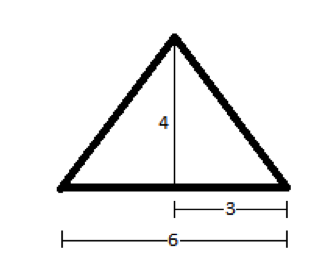### Example Question #16 : Isosceles Triangles

Two sides of a triangle each have length 6. All of the following could be the length of the third side EXCEPT

11
1
12
2
3
Explanation:

This question is about the Triangle Inequality, which states that in a triangle with two sides A and B, the third side must be greater than the absolute value of the difference between A and B and smaller than the sum of A and B.

Applying the Triangle Inequality to this problem, we see that the third side must be greater than the absolute value of the difference between the other two sides, which is |6-6|=0, and smaller than the sum of the two other sides, which is 6+6=12. The only answer choice that does not satisfy this range of possible values is 12 since the third side must be LESS than 12.

### Example Question #1 : How To Find The Area Of An Acute / Obtuse Triangle

Find the area of a triangle whose base is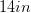and whose height is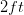.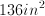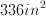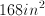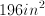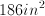Explanation:

This problem is solved using the geometric formula for the area of a triangle.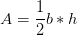Convert feet to inches.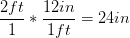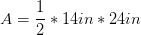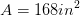### Example Question #116 : Triangles

If triangle ABC has vertices (0, 0), (6, 0), and (2, 3) in the xy-plane, what is the area of ABC?

20

12

10

18

9

9

Explanation: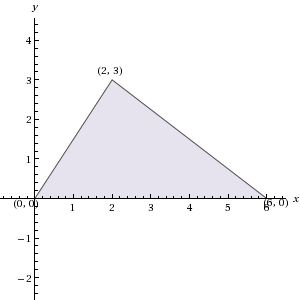Sketching ABC in the xy-plane, as pictured here, we see that it has base 6 and height 3. Since the formula for the area of a triangle is 1/2 * base * height, the area of ABC is 1/2 * 6 * 3 = 9.

### Example Question #2 : How To Find The Area Of An Acute / Obtuse Triangle

What is the area of a triangle with a height ofand a base of?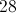Explanation:

When searching for the area of a triangle we are looking for the amount of the space enclosed by the triangle.

The equation for area of a triangle is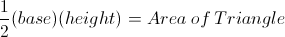Plug the values for base and height into the equation yielding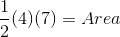Then multiply the numbers together to arrive at the answer.

### Example Question #117 : Triangles

The height,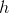, of triangle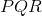in the figure is one-fourth the length of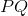. In terms of h, what is the area of triangle?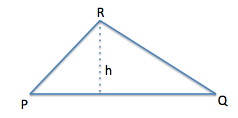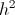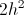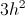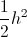Explanation:

If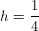*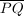, then the length ofmust be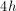.

Using the formula for the area of a triangle (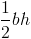), with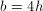, the area of the triangle must be.

### Example Question #188 : Plane Geometry

A triangle has a perimeter of 36 inches, and one side that is 12 inches long. The lengths of the other two sides have a ratio of 3:5. What is the length of the longest side of the triangle?

14

9

8

15

12

15

Explanation:

We know that the perimeter is 36 inches, and one side is 12. This means, the sum of the lengths of the other two sides are 24. The ratio between the two sides is 3:5, giving a total of 8 parts. We divide the remaining length, 24 inches, by 8 giving us 3. This means each part is 3. We multiply this by the ratio and get 9:15, meaning the longest side is 15 inches.

### Example Question #120 : Triangles

A triangle has sides of length 8, 13, and L. Which of the following cannot equal L?

9

4

6

7

15